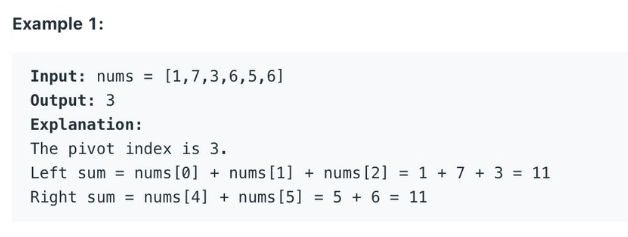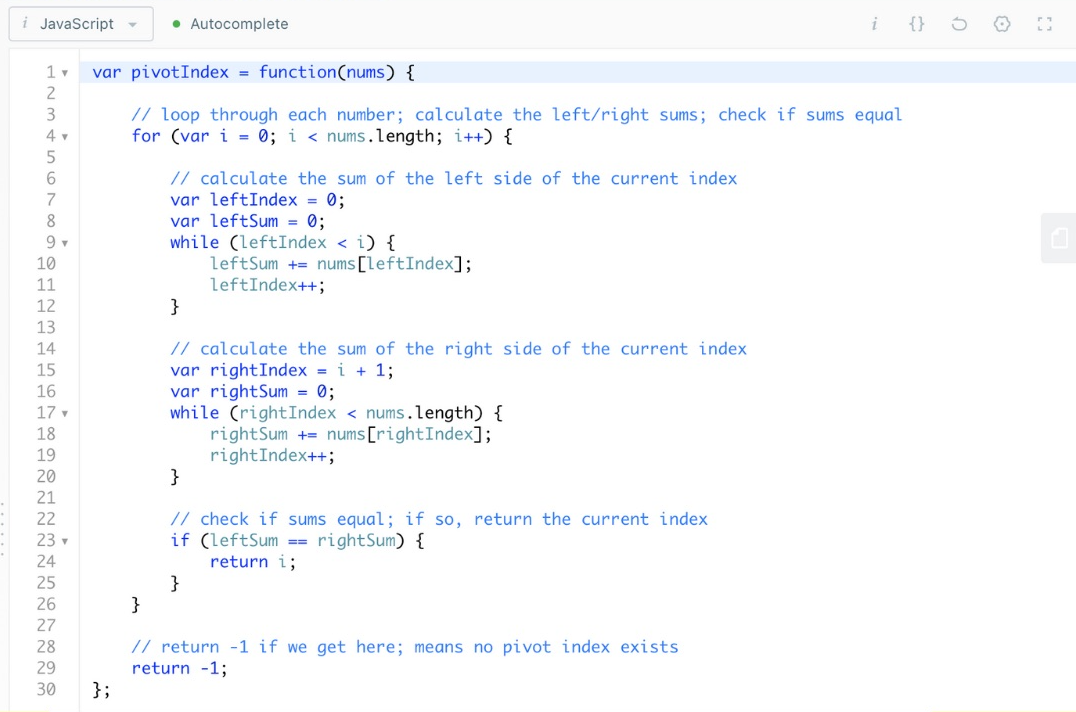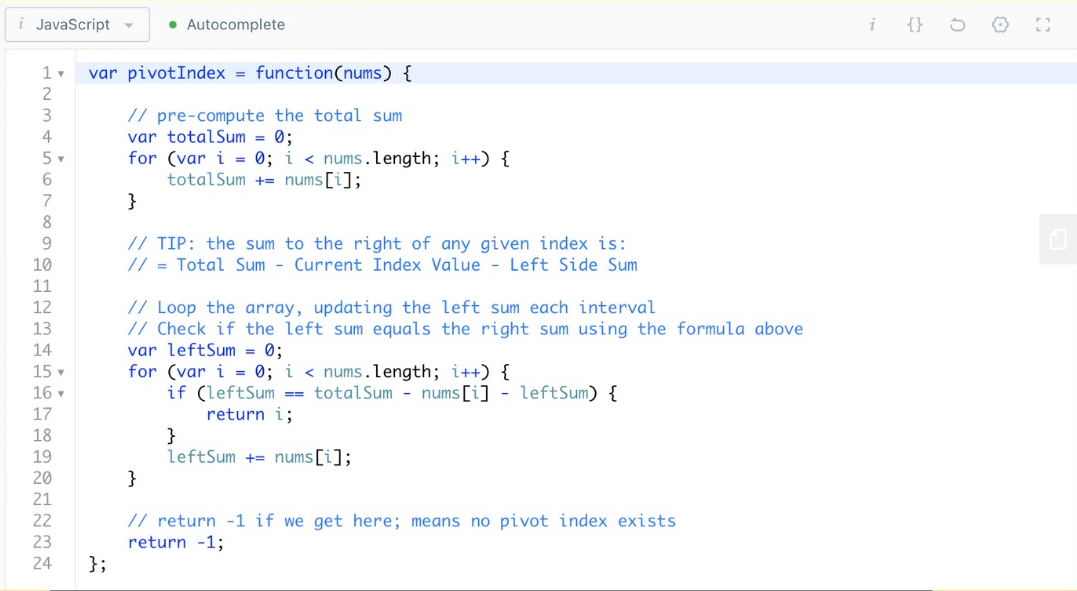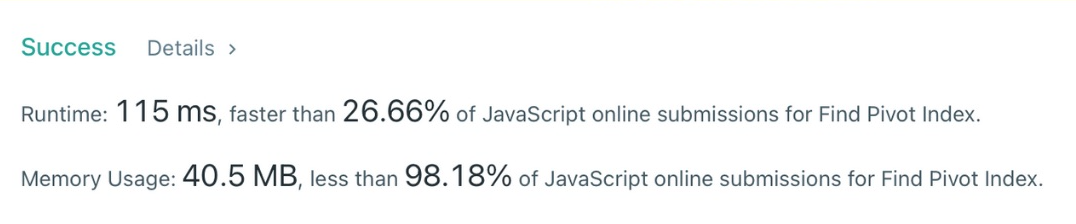# How to find the pivot index - solving the coding challenge from Leetcode!

KG.codes · 3 minute read ·

Today, we will discuss a coding challenge called Find Pivot Index from Leetcode.com. This problem presents an interesting challenge where we need to leverage pre-computing and a specific algorithm/formula to get the most optimal solution.## Problem description

"The pivot index is the index where the sum of all the numbers strictly to the left of the index is equal to the sum of all the numbers strictly to the index's right.
If the index is on the left edge of the array, then the left sum is 0 because there are no elements to the left. This also applies to the right edge of the array. Return the leftmost pivot index. If no such index exists, return -1."

Let's explore the problem further and discuss 2 different approaches to solving it.

## First try

When initially attempting to solve the problem, my first thought was to loop through the numbers, calculate the sums of the items to the left and right at each index, and then check if the sums are equal. Although this approach worked, it didn't feel optimal.I realized that this solution had a time complexity of O(n²) because for each item, I looped through its entire left and right sides (almost the entire array) in the worst case. Here, 'n' represents the length of the array. The initial loop required 'n' operations, and for each item, I performed another ~'n' operations to calculate and check the sums. This results in a total of 'n' × 'n' = 'n²' operations.## Second try

Realizing the inefficiency of my initial approach, I sought a better solution. I needed a hint, and it was that the sum to the right of any given index is equal to the total sum of the array minus the current index value minus the sum on the left side.

"the sum to the right of any given index" = Total Sum - Current Index Value - Left Side Sum

With this insight, I pre-computed the total sum of the array and then iterated through the array, updating the left sum at each interval. Simultaneously, I checked if the right sum matched the calculated value using the formula mentioned above.Implementing this new approach, I achieved the desired result. Not only did this solution work correctly, but it was also more optimal in terms of time complexity.## Time complexity

The improved solution has a time complexity of O(n). Although I looped through the array twice, I avoided nested loops as in the previous approach. Here, 'n' represents the length of the array. In the initial loop, I performed 'n' operations, and for each item, I performed another 'n' operations to calculate and check the sums. Therefore, the total number of operations is 2 × 'n' = 2n, which simplifies to O(n) which is linear time.

## Space complexity

The space complexity of the improved solution is O(1), indicating constant space usage. This is because I did not use any data structures that consume memory correlated to the size of the input array. Instead, I relied on a few variables to store intermediate results.

## Conclusion

In conclusion, I have discussed the problem of finding the pivot index in an array and presented two different approaches to solve it. The initial approach had a time complexity of O(n²), while the improved solution reduced the time complexity to O(n) without sacrificing correctness. Additionally, the improved solution achieved a space complexity of O(1), demonstrating its efficiency in terms of memory usage.

If you have any suggestions or better solutions, please let me know. I am always open to learning and improving!

Are you interested in learning more about coding challenges and improving your problem-solving skills?
Here are some useful resources:

• LeetCode - Explore LeetCode's extensive collection of coding challenges, ranging from beginner to advanced levels.
• HackerRank - Practice coding challenges and participate in coding competitions to enhance your skills.
• CodeWars - Sharpen your problem-solving abilities by completing coding challenges and engaging with a supportive community.
• InterviewBit - Prepare for coding interviews by practicing a wide range of interview questions and challenges.

Remember, consistent practice, persistence, and continuous learning are key to becoming proficient in coding challenges. Happy coding!